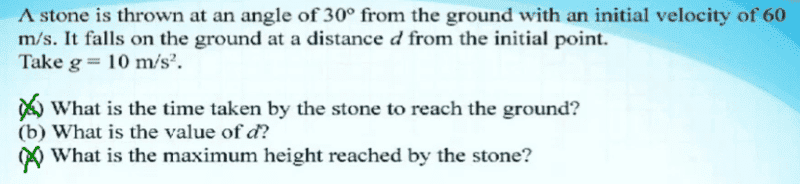# What is the distance traveled?

• prakhargupta3301

## Homework Statement

Only (b) part[/B]## Homework Equations

time of flight= (2⋅u⋅sinθ)/g ---------------- - 1
total distance (range)= u⋅cosθ * time of flight (s=vt)
=u⋅cosθ * (2⋅u⋅sinθ)/g --------------- -2
=u2⋅2sinθcosθ/g
= u2⋅sin2θ/g ---------------- -3

## The Attempt at a Solution

[/B]
Rather than using equation 3, I decided to use 2, as 3 is just 2 simplified.
Distance= u⋅cosθ * (2⋅u⋅sinθ)/g
= (60*cos30°)*(2*60*sin30°)/10
= (60*(√3/2))*(2*60*(1/2))/10
=(30*60√3)/10
=180√3 metres

However by equation 3,
Distance= u2⋅sin2θ/g
= 3600*(sin30°)2/10
= [3600*(1/4)]/10
= 900/10= 90 metres.
Where is the error please tell me!

#### Attachments

Last edited:
2sinxcosx = sin(2x) whereas you are using sin2(x)
You should get 180√3 either way.

•prakhargupta3301
Thanks a bunch. I redid the mistake over and over again.
Now it's clear.
:D